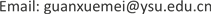1. 引言

2. 电磁力计算模型

3. 空气隙中的电磁力

1) 在电磁蜗杆、蜗轮和气隙中磁场是分段均匀的。

2) 铁心的磁感应强度不能高于其饱和磁感应强度，即处于不饱和状态。

3) 假设磁通全部流过铁心，不考虑漏磁和磁滞的影响。

4) 蜗杆上的线圈和电源之间没有电能交换。

5) 忽略铁芯磁阻误差。

f = B 0 2 A q μ 0 (1)

N i = ∮ H d s = L c l H 2 + 2 L q H 0 (2)

f i = ( N i L c l μ + 2 L q μ 0 ) 2 A q μ 0 = ( N i L c l μ 2 r + 2 L q ) 2 μ 0 A q (3)

f i = ( N i 2 L q ) 2 μ 0 A q = i 2 L 2 q N 2 μ 0 A q 4 = k i 2 L 2 q

Parameters summary shee

N = 205α1 = −45˚ α2 = −27˚ α3 = −9˚ α4 = 9˚ α5 = 27˚
N = 184.5α1 = −45˚ α2 = −25˚ α3 = −5˚ α4 = 13˚ α5 = 33˚
N = 164α1 = −45˚ α2 = −23˚ α3 = 0˚ α4 = 23˚
N = 144α1 = −45˚ α2 = −19˚ α3 = 7˚ α4 = 33˚
N = 123α1 = −45˚ α2 = −15˚ α3 = 15˚
N = 103α1 = −45˚ α2 = −9˚ α3 = 27˚
N = 82α1 = −45˚ α2 = 0˚
N = 62α1 = −45˚ α2 = 15˚
N = 41α1 = −45˚

1) 不同磁极的电磁力分布图形大致一样，当蜗轮磁极数增加时，其啮合磁极数也增加，因而电磁力大小也随着增大。

2) 影响电磁力大小的主要有蜗杆线圈的通电电流和空气隙。电磁力的大小主要受空气隙的影响比较大，蜗杆线圈的通电电流对电磁力的影响在其取值小的时候比较明显，取值大时则对电磁力影响不大。

3) 当空气隙由0.2 mm增加到0.4 mm时，电磁力迅速减小，当空气隙由0.4 mm增加到1 mm时，电磁力缓慢减小。

4) 当通电电流由0.5 A增加到1.5 A时，电磁力随着电流的增加而迅速增加，当通电电流由1.5 A增加到5 A时，电磁力增加的非常缓慢。

4. 系统输出力矩

T n = f q R

The output torque of the syste

5. 电磁蜗杆传动二维有限元分析

1) 从磁力线分布图上可以得出：磁通流线密的地方表示该处磁感应强度大，流线稀疏的地方表示该处磁感应强度弱，并且和磁感应强度分布规律相对应，磁通流线主要集中分布于磁体附近，并且以永磁体径向中心线为对称分布，在中心线最外侧处磁通流线最密。

2) 从磁感应强度分布图中可以看到：永磁体附近的磁感应强度数值大约在0.2 T~1.5 T左右。其中有黄线和红线的地方，表明该处的场强较强，而出现场强较强的地方主要在两块永磁体交界处，这是由于在角点处没有做钝化处理的结果。磁感应强度在稍远于永磁体附近的区域中是蓝色区域，表明场强较弱。在磁体外侧边缘的区域中磁感应强度大多为绿色和黄色，表明该处的场强较强，这也是电磁蜗杆传动的工作区，为了避免磁场强度衰减过快，蜗轮与蜗杆之间气隙尽量小。

3) 通过不同充磁方式情况下磁感应强度最高值和最低值比较来看：径向切向交错充磁与径向充磁的最高值相差不多，总体上是径向充磁数值高点；径向切向交错充磁时，不同极数其最小磁感应强度值相差很多，最少相差几十倍，最多相差几万倍，因此在比较图上舍去了最大数值(N = 4、N = 6时)，径向充磁的最小值比径向切向交错充磁最小值小的很多。

4) 从漏磁区的磁力线分布可以看出：随着永磁体个数的增加，漏磁区的范围减小，因此在系统设计时蜗轮尽可能采用较多的永磁体数，较多的永磁体数也有利于增加蜗轮和蜗杆的啮合齿数，从而增加力矩输出。

6. 结论

1) 为了提高永磁体的利用效率，必须尽量减小气隙，气隙控制在1 mm范围为好。随着磁极数增加，磁漏区范围也越小，所以实际应用中要尽可能采用数量多的磁极。随着磁极数增加，每个磁极的磁通密度也增加，因而传递的力矩也越大。

2) 为避免磁场强度衰减过快，蜗轮与蜗杆之间气隙应该尽量小，气隙控制在1 mm范围为好。

3) 电磁蜗杆传动工作区域是永磁体外侧，内侧不是工作区，不用考虑最小值取值的蓝色区域，综合考虑永磁体采用径向充磁方式。

4) 系统设计时蜗轮尽可能采用较多的永磁体数。